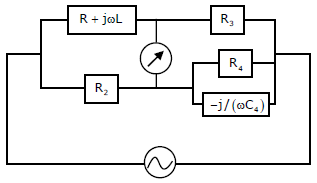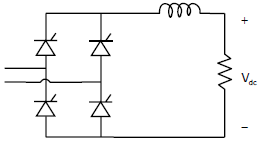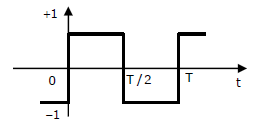# EE GATE 2010 - Online Test

Q1. The Maxwell’s bridge shown in the figure is at balance. The parameters of the inductive coil areExplaination / Solution:
No Explaination.

Q2. Assuming that the diodes in the given circuit are ideal, the voltage V0 isExplaination / Solution:
No Explaination.

Q3.
The power electronic converter shown in the figure has a single-pole double-throw switch. The pole P of the switch is connected alternately to throws A and B. The converter shown is a _______Explaination / Solution:
No Explaination.

Q4.
Figure shows a composite switch consisting of a power transistor (BJT) in series with a diode. Assuming that the transistor switch and the diode are ideal , the I-V characteristic of the composite switch isExplaination / Solution:
No Explaination.

Q5.
The fully controlled thyristor converter in the figure is fed from a single-phase source. When the firing angle is 0°, the dc output voltage of the converter is 300V. What will be the output voltage for a firing angle of 60°, assuming continuous conduction?Explaination / Solution:
No Explaination.

Q6.
The second harmonic component of the periodic waveform given in the figure has an amplitude ofExplaination / Solution:
No Explaination.

Q7. The transistor circuit shown uses a silicon transistor with VBE = 0.7V, IC ≈ IE and a dc current gain of 100. The value of V0 isExplaination / Solution:
No Explaination.

Q8.
The TTL circuit shown in the figure is fed with the waveform X (also shown). All gates have equal propagation delay of 10ns. The output Y of the circuit isExplaination / Solution:
No Explaination.

Q9. When a “CALL Addr” instruction is executed, the CPU carries out the following sequential operations internally: Note: (R) means content of register R ((R)) means content of memory location pointed to by R PC means Program Counter SP means Stack Pointer
Explaination / Solution:
No Explaination.

Q10. The following Karnaugh map represent a function F.A minimized form of the function F is
Explaination / Solution:
No Explaination.